# Definition Variables, Matrix Specification¶

This example will demonstrate the use of OpenMx definition variables with the implementation of a simple two group dataset. What are definition variables? Essentially, definition variables can be thought of as observed variables which are used to change the statistical model on an individual case basis. In essence, it is as though one or more variables in the raw data vectors are used to specify the statistical model for that individual. Many different types of statistical models can be specified in this fashion; some can be readily specified in standard fashion, and some that cannot. To illustrate, we implement a two-group model. The groups differ in their means but not in their variances and covariances. This situation could easily be modeled in a regular multiple group fashion - it is only implemented using definition variables to illustrate their use. The results are verified using summary statistics and an Mx 1.0 script for comparison is also available.

## Mean Differences¶

The example shows the use of definition variables to test for mean differences. It is available in the following file:

A parallel version of this example, using path specification of models rather than matrices, can be found here:

### Statistical Model¶

Algebraically, we are going to fit the following model to the observed x and y variables: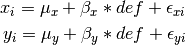where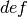is the definition variable and the residual sources of variance,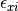and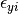covary to the extent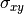. So, the task is to estimate: the two means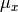and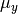; the deviations from these means due to belonging to the group identified by havingset to 1 (as opposed to zero),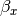and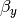; and the parameters of the variance covariance matrix: cov(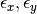) =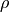.

Our task is to implement the model shown in the figure below: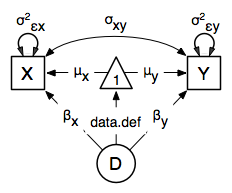### Data Simulation¶

Our first step to running this model is to simulate the data to be analyzed. Each individual is measured on two observed variables, x and y, and a third variable def which denotes their group membership with a 1 or a 0. These values for group membership are not accidental, and must be adhered to in order to obtain readily interpretable results. Other values such as 1 and 2 would yield the same model fit, but would make the interpretation more difficult.

library(MASS)  # to get hold of mvrnorm function
set.seed(200)
N              <- 500
Sigma          <- matrix(c(1,.5,.5,1),2,2)
group1         <- mvrnorm(N, c(1,2), Sigma) # Use mvrnorm from MASS package
group2         <- mvrnorm(N, c(0,0), Sigma)


We make use of the superb R function mvrnorm in order to simulate N=500 records of data for each group. These observations correlate .5 and have a variance of 1, per the matrix Sigma. The means of x and y in group 1 are 1.0 and 2.0, respectively; those in group 2 are both zero. The output of the mvrnorm function calls are matrices with 500 rows and 3 columns, which are stored in group 1 and group 2. Now we create the definition variable

# Put the two groups together, create a definition variable,
# and make a list of which variables are to be analyzed (selVars)
xy             <- rbind(group1,group2)      # Bind groups together by rows
def            <- rep(c(1,0),each=N);       # Add def var [2n] for group status
selVars        <- c("x","y")                # Make selection variables object


The objects xy and def might be combined in a data frame. However, in this case we won’t bother to do it externally, and simply paste them together in the mxData function call.

### Model Specification¶

The following code contains all of the components of our model. Before running a model, the OpenMx library must be loaded into R using either the require() or library() function. This code uses the mxModel function to create an mxModel object, which we’ll then run. Note that all the objects required for estimation (data, matrices, and an objective function) are declared within the mxModel function. This type of code structure is recommended for OpenMx scripts generally.

dataRaw      <- mxData( observed=data.frame(xy,def), type="raw" )
# covariance matrix
Sigma        <- mxMatrix( type="Symm", nrow=2, ncol=2,
free=TRUE, values=c(1, 0, 1), name="Sigma" )
# means
Mean         <- mxMatrix( type="Full", nrow=1, ncol=2,
free=TRUE, name="Mean" )
# regression coefficient
beta         <- mxMatrix( type="Full", nrow=1, ncol=2,
free=TRUE, values=c(0,0), name="beta" )
# definition variable
dataDef      <- mxMatrix( type="Full", nrow=1, ncol=2,
free=FALSE, labels=c("data.def"), name="def" )
Mu           <- mxAlgebra( expression=Mean + beta*def, name="Mu" )
exp          <- mxExpectationNormal( covariance="Sigma", means="Mu", dimnames=selVars )
funML        <- mxFitFunctionML()

defMeansModel <- mxModel("Definition  Means Matrix Specification",
dataRaw, Sigma, Mean, beta, dataDef, Mu, exp, funML)


The first argument in an mxModel function has a special purpose. If an object or variable containing an MxModel object is placed here, then mxModel adds to or removes pieces from that model. If a character string (as indicated by double quotes) is placed first, then that becomes the name of the model. Models may also be named by including a name argument. This model is named "Definition Means Matrix Specification".

Next, we declare where the data are, and their type, by creating an MxData object with the mxData function. This piece of code creates an MxData object. It first references the object where our data are, then uses the type argument to specify that this is raw data. Because the data are raw and the fit function is mxFitFunctionML, full information maximum likelihood is used in this mxModel. Analyses using definition variables have to use raw data, so that the model can be specified on an individual data vector level.

dataRaw      <- mxData( observed=data.frame(xy,def), type="raw" )


Model specification is carried out using mxMatrix functions to create matrices for the model. In the present case, we need four matrices. First is the predicted covariance matrix, Sigma. Next, we use three matrices to specify the model for the means. First is Mean which corresponds to estimates of the means for individuals with definition variables with values of zero. Individuals with definition variable values of 1 will have the value in Mean plus the value in the matrix beta. So both matrices are of size 1x2 and both contain two free parameters. There is a separate deviation for each of the variables, which will be estimated in the elements 1,1 and 1,2 of the beta matrix. Last, but by no means least, is the matrix def which contains the definition variable. The variable def in the mxData data frame is referred to in the matrix label as data.def. In the present case, the definition variable contains a 1 for group 1, and a zero otherwise.

The trick - commonly used in regression models - is to multiply the beta matrix by the def matrix. This multiplication is effected using an mxAlgebra function call:

beta         <- mxMatrix( type="Full", nrow=1, ncol=2,
free=TRUE, values=c(0,0), name="beta" )
dataDef      <- mxMatrix( type="Full", nrow=1, ncol=2,
free=FALSE, labels=c("data.def"), name="def" )
Mu           <- mxAlgebra( expression=Mean + beta*def, name="Mu" )


The result of this algebra is named Mu, and this handle is referred to in the mxExpectationNormal function call.

The last argument in this mxModel call is itself a function. It declares that the fit function to be optimized is maximum likelihood (ML), which is tagged mxFitFunctionML. Full information maximum likelihood (FIML) is used whenever the data allow, and does not need to be requested specifically. The third argument in this mxModel is another function. It declares the expectation function to be a normal distribution, mxExpectationNormal. This means the model is of a normal distribution with a particular mean and covariance. Hence, there are in turn two arguments to this function: the covariance matrix Sigma and the mean vector Mu. These matrices will be defined later in the mxModel function call.

mxFitFunctionML()
mxExpectationNormal( covariance="Sigma", means="Mu", dimnames=selVars )


We can then run the model and examine the output with a few simple commands.

### Model Fitting¶

# Run the model
defMeansFit <- mxRun(defMeansModel)
defMeansFit$matrices defMeansFit$algebras


It is possible to compare the estimates from this model to some summary statistics computed from the data:

# Compare OpenMx estimates to summary statistics computed from raw data.
# Note that to calculate the common variance,
# group 1 has 1 and 2 subtracted from every Xi and Yi in the sample data,
# so as to estimate variance of combined sample without the mean correction.

# First compute some summary statistics from data
ObsCovs        <- cov(rbind(group1 - rep(c(1,2),each=N), group2))
ObsMeansGroup1 <- c(mean(group1[,1]), mean(group1[,2]))
ObsMeansGroup2 <- c(mean(group2[,1]), mean(group2[,2]))

# Second extract parameter estimates and matrix algebra results from model
Sigma          <- mxEval(Sigma, defMeansFit)
Mu             <- mxEval(Mu, defMeansFit)
Mean           <- mxEval(Mean, defMeansFit)
beta           <- mxEval(beta, defMeansFit)

# Third, check to see if things are more or less equal
omxCheckCloseEnough(ObsCovs,Sigma,.01)
omxCheckCloseEnough(ObsMeansGroup1,as.vector(Mean+beta),.001)
omxCheckCloseEnough(ObsMeansGroup2,as.vector(Mean),.001)


These models may also be specified using paths instead of matrices. See Definition Variables, Path Specification for path specification of these models.